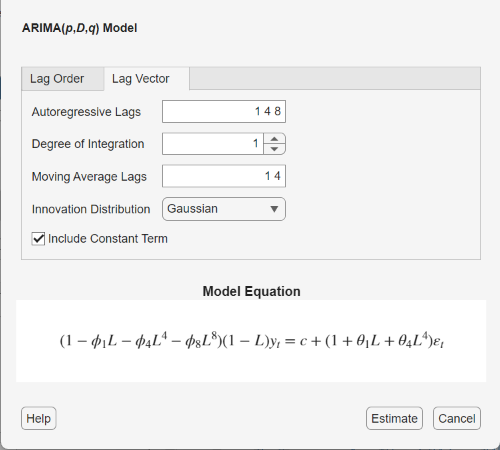Main Content

## ARIMA Model Specifications

### Default ARIMA Model

This example shows how to use the shorthand `arima(p,D,q)` syntax to specify the default ARIMA(p, D, q) model,

`${\Delta }^{D}{y}_{t}=c+{\varphi }_{1}{\Delta }^{D}{y}_{t-1}+\dots +{\varphi }_{p}{\Delta }^{D}{y}_{t-p}+{\epsilon }_{t}+{\theta }_{1}{\epsilon }_{t-1}+\dots +{\theta }_{q}{\epsilon }_{t-q},$`

where ${\Delta }^{D}{y}_{t}$ is a ${D}^{th}$ differenced time series. You can write this model in condensed form using lag operator notation:

`$\varphi \left(L\right)\left(1-L{\right)}^{D}{y}_{t}=c+\theta \left(L\right){\epsilon }_{t}.$`

By default, all parameters in the created model object have unknown values, and the innovation distribution is Gaussian with constant variance.

Specify the default ARIMA(1,1,1) model:

`Mdl = arima(1,1,1)`
```Mdl = arima with properties: Description: "ARIMA(1,1,1) Model (Gaussian Distribution)" Distribution: Name = "Gaussian" P: 2 D: 1 Q: 1 Constant: NaN AR: {NaN} at lag  SAR: {} MA: {NaN} at lag  SMA: {} Seasonality: 0 Beta: [1×0] Variance: NaN ```

The output shows that the created model object, `Mdl`, has `NaN` values for all model parameters: the constant term, the AR and MA coefficients, and the variance. You can modify the created model using dot notation, or input it (along with data) to `estimate`.

The property `P` has value 2 (p + D). This is the number of presample observations needed to initialize the AR model.

### ARIMA Model with Known Parameter Values

This example shows how to specify an ARIMA(p, D, q) model with known parameter values. You can use such a fully specified model as an input to `simulate` or `forecast`.

Specify the ARIMA(2,1,1) model

`$\Delta {y}_{t}=0.4+0.8\Delta {y}_{t-1}-0.3\Delta {y}_{t-2}+{\epsilon }_{t}+0.5{\epsilon }_{t-1},$`

where the innovation distribution is Student's t with 10 degrees of freedom, and constant variance 0.15.

```tdist = struct('Name','t','DoF',10); Mdl = arima('Constant',0.4,'AR',{0.8,-0.3},'MA',0.5,... 'D',1,'Distribution',tdist,'Variance',0.15)```
```Mdl = arima with properties: Description: "ARIMA(2,1,1) Model (t Distribution)" Distribution: Name = "t", DoF = 10 P: 3 D: 1 Q: 1 Constant: 0.4 AR: {0.8 -0.3} at lags [1 2] SAR: {} MA: {0.5} at lag  SMA: {} Seasonality: 0 Beta: [1×0] Variance: 0.15 ```

The name-value pair argument `D` specifies the degree of nonseasonal integration (D).

All parameter values are specified, that is, no object property is `NaN`-valued.

### Specify ARIMA Model Using Econometric Modeler App

In the Econometric Modeler app, you can specify the lag structure, presence of a constant, and innovation distribution of an ARIMA(p,D,q) model by following these steps. All specified coefficients are unknown but estimable parameters.

1. At the command line, open the Econometric Modeler app.

`econometricModeler`

Alternatively, open the app from the apps gallery (see Econometric Modeler).

2. In the Time Series pane, select the response time series to which the model will be fit.

3. On the Econometric Modeler tab, in the Models section, click . To create ARIMAX models, see ARIMAX Model Specifications.

The ARIMA Model Parameters dialog box appears.4. Specify the lag structure. To specify an ARIMA(p,D,q) model that includes all AR lags from 1 through p and all MA lags from 1 through q, use the Lag Order tab. For the flexibility to specify the inclusion of particular lags, use the Lag Vector tab. For more details, see Specifying Lag Operator Polynomials Interactively. Regardless of the tab you use, you can verify the model form by inspecting the equation in the Model Equation section.

For example:

• To specify an ARIMA(3,1,2) model that includes a constant, includes all consecutive AR and MA lags from 1 through their respective orders, and has a Gaussian innovation distribution:

1. Set Degree of Integration to `1`.

2. Set Autoregressive Order to `3`.

3. Set Moving Average Order to `2`.

• To specify an ARIMA(3,1,2) model that includes all AR and MA lags from 1 through their respective orders, has a Gaussian distribution, but does not include a constant:

1. Set Degree of Integration to `1`.

2. Set Autoregressive Order to `3`.

3. Set Moving Average Order to `2`.

4. Clear the Include Constant Term check box.

• To specify an ARIMA(8,1,4) model containing nonconsecutive lags

`$\left(1-{\varphi }_{1}L-{\varphi }_{4}{L}^{4}-{\varphi }_{8}{L}^{8}\right)\left(1-L\right){y}_{t}=\left(1+{\theta }_{1}{L}^{1}+{\theta }_{4}{L}^{4}\right){\epsilon }_{t},$`

where εt is a series of IID Gaussian innovations:

1. Click the Lag Vector tab.

2. Set Degree of Integration to `1`.

3. Set Autoregressive Lags to `1 4 8`.

4. Set Moving Average Lags to `1 4`.

5. Clear the Include Constant Term check box.• To specify an ARIMA(3,1,2) model that includes all consecutive AR and MA lags through their respective orders and a constant term, and has t-distribution innovations:

1. Set Degree of Integration to `1`.

2. Set Autoregressive Order to `3`.

3. Set Moving Average Order to `2`.

4. Click the button, then select `t`.

The degrees of freedom parameter of the t distribution is an unknown but estimable parameter.

After you specify a model, click to estimate all unknown parameters in the model.

Download ebook